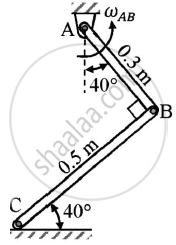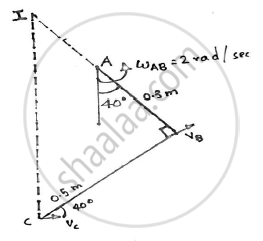Advertisement Remove all ads

# A Rod Ab Has an Angular Velocity of 2 Rad/Sec,Counter Clock Wise as Shown.End C of Rod Bc is Free to Move on a Horizontal Surface.Determine: (1)Angular Velocity of Rod Bc (2)Velocity of C - Engineering Mechanics

Answer in Brief

A rod AB has an angular velocity of 2 rad/sec,counter clock wise as shown.End C of rod BC is free to move on a horizontal surface.Determine:
(1)Angular velocity of rod BC
(2)Velocity of CGiven : ωAB=2 rad/sec (anti clockwise)

To find : ωBC
VC

Advertisement Remove all ads

#### SolutionBY GEOMETRY :
Assume I to be the ICR of rod BC

In △IAB,

∠BIC=40°

∠IBC=90°
tan 40 = (BC)/(IB) = (0.5)/(IB)

sin 40 = (BC)/(IC)  = (0.5)/(IC)
IB = 0.5959m and IC = 0.7779m

vB = rω

= ABxωAB
= 0.3 x 2
= 0.6 m/s

ωBC = (v_B)/r
= (v_B)/(IB)
= (0.6)/(0.5959)
=1.0069 rad/sec
The direction is anti-clockwise

vC = rω

= IC x ωBC
= 0.7779 x 1.0069
= 0.7832 m/s
The direction of vC is towards right

Angular velocity of BC=1.0069 rad/sec(anto clockwise)
vC=0.7832 m/s(Towards right)

Concept: Velocity and Acceleration in Terms of Rectangular Co-ordinate System
Is there an error in this question or solution?
Advertisement Remove all ads

#### APPEARS IN

Advertisement Remove all ads
Advertisement Remove all ads
Share
Notifications

View all notifications

Forgot password?